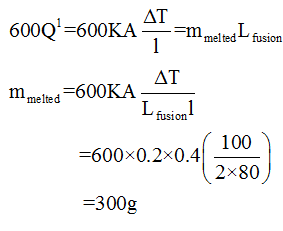# The opposite faces of a cubical block of iron of cross section 4cm2 are kept in contact with steam and melting ice. Calculate the quantity of ice melted at the end of 10min. Given k for iron=0.2cal/s cm C degree.

Question
2 views

The opposite faces of a cubical block of iron of cross section 4cm2 are kept in contact with steam and melting ice. Calculate the quantity of ice melted at the end of 10min. Given k for iron=0.2cal/s cm C degree.

check_circle

Step 1

The heat transfer equation is given by

Step 2

The heat flows for 10min, i.e. heat absorbed by ice .

Step 3

This heat flow is equal to the latent heat of fusion times the mass of melted ice....

### Want to see the full answer?

See Solution

#### Want to see this answer and more?

Solutions are written by subject experts who are available 24/7. Questions are typically answered within 1 hour.*

See Solution
*Response times may vary by subject and question.
Tagged in

### Thermodynamics